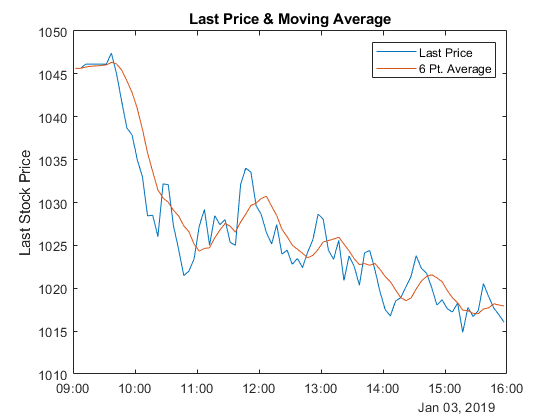# Visualize Simple Moving Average of Your Data

This example shows how to calculate the simple moving average of a stock price over time and visualize the results.

ThingSpeak™ channel 276806 contains delayed financial data. The data is collected once every five minutes. The first six fields contain price and number data for the last, high, low, volume, open, and close values, respectively. Read the data using the `thingSpeakRead` function from the channel 276806 on a particular day, for example, January 4, 2018.

```data = thingSpeakRead(276806,'DateRange',[datetime('January 3, 2019 0:0:0') datetime('January 4, 2019 0:0:0')],'Fields',1,'outputFormat','timetable'); ```

### Calculate the Simple Moving Average

Use the `movavg` function to calculate the simple moving average. Set the lag as 6, which indicates the window size or number of periods for the moving average. The window size of 6 represents 30 minutes of data. The default behavior for `movavg` is unweighted, or a simple moving average.

```lag = 6; simple = movavg(data.Last,'simple',lag); ```

### Plot the Moving Average

Plot the simple moving average calculations for stock price values over time.

```plot(data.Timestamps,data.Last, data.Timestamps,simple); legend('Last Price','6 Pt. Average'); ylabel('Last Stock Price'); title('Last Price & Moving Average'); ```The plot shows the share price and the moving average of the share price over the course of a day.